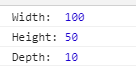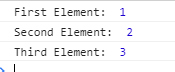# What is the use of curly brackets in the `var { … } = …` statements ?

Destructuring assignment allows us to assign the properties of an array or object to a bunch of variables that are sometimes very convenient and short. Consider the following illustration. Both of the below-mentioned methods are right and produce the same result.

• Without Destructuring:
```var array = [1, 20, 40];

var first = array
var second = array
var third = arr
```
• With Destructuring
```var array = [1, 20, 40];

var [first, second, third] = array;
```

Object Destructuring Destructuring can be done on JavaScript objects. On the left side of the assignment operator, there is a pattern of variables in which the properties of an object are to be stored. The variable’s name must be the same as defined in the object. Let’s have a look at this concept from the following example.

Note: Curly brackets ‘{ }’ are used to destructure the JavaScript Object properties.

• Example:

 ` ` `<``html` `lang``=``"en"``> ` ` `  `<``head``> ` `    ``<``meta` `charset``=``"UTF-8"``> ` `    ``<``title``>GeeksForGeeks ` ` ` ` `  `<``body``> ` `    ``<``script``> ` `     `  `        ``let example_object = { ` `            ``name: "Object", ` `            ``platform: "GeeksForGeeks", ` `            ``number: 100 ` `        ``}; ` `     `  `        ``let {name, platform, number} = example_object; ` `     `  `        ``console.log("Name: ", name); ` `        ``console.log("Platform: ", platform); ` `        ``console.log("Number: ", number); ` `    `` ` ` ` ` `  ` `

• Output:If we want the variables defined in the object should be assigned to the variables with other names then we can set it using a colon.

• Syntax:
`{sourceProperty : targetVariable}`
• Example:

 ` ` `<``html` `lang``=``"en"``> ` ` `  `<``head``> ` `    ``<``meta` `charset``=``"UTF-8"``> ` `    ``<``title``>GeeksForGeeks ` ` ` ` `  `<``body``> ` `    ``<``script``> ` `        ``let cuboid = { ` `            ``width: 100, ` `            ``height: 50, ` `            ``depth: 10 ` `        ``}; ` `     `  `        ``// width -> w ` `        ``// height -> h ` `        ``// depth -> d ` `        ``let {width:w, height:h, depth:d} = cuboid; ` `     `  `        ``console.log("Width: ", w); ` `        ``console.log("Height:", h); ` `        ``console.log("Depth: ", d); ` `    `` ` ` ` ` `  ` `

• Output:Array Destructuring: The elements of the array can also be destructured in the same way. The destructuring assignment can be used to assign the array values to a bunch of different variables in JavaScript.

Note: Square brackets ‘[ ]’ are used to destructure the array elements.

• Example:

 ` ` `<``html` `lang``=``"en"``> ` ` `  `<``head``> ` `    ``<``meta` `charset``=``"UTF-8"``> ` `    ``<``title``>GeeksForGeeks ` ` ` ` `  `<``body``> ` `    ``<``script``> ` `        ``var arr = [1, 2, 3]; ` `     `  `        ``var [arr_1, arr_2, arr_3] = arr; ` `     `  `        ``console.log("First Element: ", arr_1); ` `        ``console.log("Second Element: ", arr_2); ` `        ``console.log("Third Element: ", arr_3); ` `    `` ` ` ` ` `  ` `

• Output:My Personal Notes arrow_drop_upCheck out this Author's contributed articles.

If you like GeeksforGeeks and would like to contribute, you can also write an article using contribute.geeksforgeeks.org or mail your article to contribute@geeksforgeeks.org. See your article appearing on the GeeksforGeeks main page and help other Geeks.

Please Improve this article if you find anything incorrect by clicking on the "Improve Article" button below.